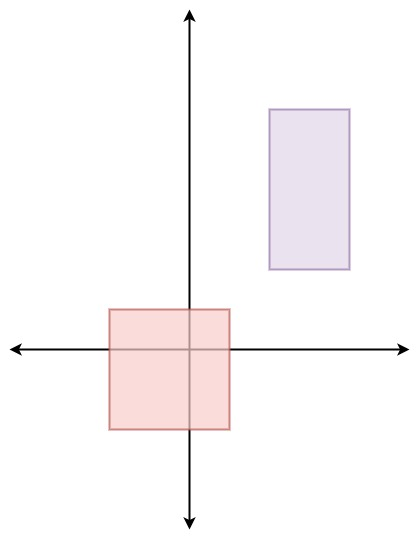## Algorithm

Problem Name: 497. Random Point in Non-overlapping Rectangles

You are given an array of non-overlapping axis-aligned rectangles `rects` where `rects[i] = [ai, bi, xi, yi]` indicates that `(ai, bi)` is the bottom-left corner point of the `ith` rectangle and `(xi, yi)` is the top-right corner point of the `ith` rectangle. Design an algorithm to pick a random integer point inside the space covered by one of the given rectangles. A point on the perimeter of a rectangle is included in the space covered by the rectangle.

Any integer point inside the space covered by one of the given rectangles should be equally likely to be returned.

Note that an integer point is a point that has integer coordinates.

Implement the `Solution` class:

• `Solution(int[][] rects)` Initializes the object with the given rectangles `rects`.
• `int[] pick()` Returns a random integer point `[u, v]` inside the space covered by one of the given rectangles.

Example 1:```Input
["Solution", "pick", "pick", "pick", "pick", "pick"]
[[[[-2, -2, 1, 1], [2, 2, 4, 6]]], [], [], [], [], []]
Output
[null, [1, -2], [1, -1], [-1, -2], [-2, -2], [0, 0]]

Explanation
Solution solution = new Solution([[-2, -2, 1, 1], [2, 2, 4, 6]]);
solution.pick(); // return [1, -2]
solution.pick(); // return [1, -1]
solution.pick(); // return [-1, -2]
solution.pick(); // return [-2, -2]
solution.pick(); // return [0, 0]
```

Constraints:

• `1 <= rects.length <= 100`
• `rects[i].length == 4`
• `-109 <= ai < xi <= 109`
• `-109 <= bi < yi <= 109`
• `xi - ai <= 2000`
• `yi - bi <= 2000`
• All the rectangles do not overlap.
• At most `104` calls will be made to `pick`.

## Code Examples

### #1 Code Example with Javascript Programming

```Code - Javascript Programming```

``````
const Solution = function(rects) {
this.rects = rects
this.areas = rects.map(([x1, y1, x2, y2]) => (x2 - x1 + 1) * (y2 - y1 + 1))
}

Solution.prototype.pick = function() {
const { rects, areas } = this
let areaSum = 0
let selected
for (let i = 0; i < rects.length; i++) {
const area = areas[i]
areaSum += area
const p = area / areaSum
if (Math.random() < p) {
selected = rects[i]
}
}
const [x1, y1, x2, y2] = selected
return [
((Math.random() * (x2 - x1 + 1)) | 0) + x1,
((Math.random() * (y2 - y1 + 1)) | 0) + y1
]
}
``````
Copy The Code &

Input

cmd
["Solution", "pick", "pick", "pick", "pick", "pick"] [[[[-2, -2, 1, 1], [2, 2, 4, 6]]], [], [], [], [], []]

Output

cmd
[null, [1, -2], [1, -1], [-1, -2], [-2, -2], [0, 0]]

### #2 Code Example with Python Programming

```Code - Python Programming```

``````
class Solution:

def __init__(self, rects):
self.rects, self.ranges, sm = rects, [], 0
for x1, y1, x2, y2 in rects:
sm += (x2 - x1 + 1) * (y2 - y1 + 1)
self.ranges.append(sm)

def pick(self):
x1, y1, x2, y2 = self.rects[bisect.bisect_left(self.ranges, random.randint(1, self.ranges[-1]))]
return [random.randint(x1, x2), random.randint(y1, y2)]
``````
Copy The Code &

Input

cmd
["Solution", "pick", "pick", "pick", "pick", "pick"] [[[[-2, -2, 1, 1], [2, 2, 4, 6]]], [], [], [], [], []]

Output

cmd
[null, [1, -2], [1, -1], [-1, -2], [-2, -2], [0, 0]]

### #3 Code Example with C# Programming

```Code - C# Programming```

``````
using System;

namespace LeetCode
{
public class _0497_RandomPointInNonOverlappingRectangles
{

public _0497_RandomPointInNonOverlappingRectangles(int[][] rects)
{
this.rects = rects;
points = new int[rects.Length];
for (int i = 0; i < rects.Length; i++)
{
var rect = rects[i];
totalPointCount += (rect - rect + 1) * (rect - rect + 1);
points[i] = totalPointCount;
}

random = new Random();
}

public int[] Pick()
{
var target = random.Next(totalPointCount);
var index = Array.BinarySearch(points, target);
if (index < 0)
index = ~index;
else
index++;

var rect = rects[index];
var width = rect - rect + 1;
var height = rect - rect + 1;
var prevPoints = points[index] - width * height;
return new int { rect + (target - prevPoints) % width, rect + (target - prevPoints) / width };
}
}

}
``````
Copy The Code &

Input

cmd
["Solution", "pick", "pick", "pick", "pick", "pick"] [[[[-2, -2, 1, 1], [2, 2, 4, 6]]], [], [], [], [], []]

Output

cmd
[null, [1, -2], [1, -1], [-1, -2], [-2, -2], [0, 0]]